# b) i. Using e-8 definition show that f is continuous at (0,0), where f(x,y) = {aš...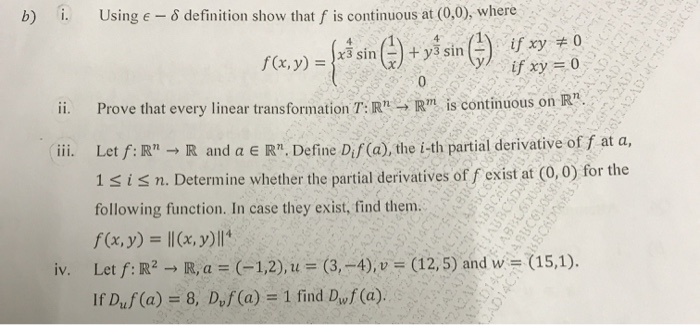b) i. Using e-8 definition show that f is continuous at (0,0), where f(x,y) = {aš sin () + yś sin () if xy + 0 242ADES if xy = 0 ii. Prove that every linear transformation T:R" - R" is continuous on R". iii. Let f:R" → R and a ER" Define Dis (a), the i-th partial derivative of f at a, 1 sisn. Determine whether the partial derivatives of f exist at (0,0) for the following function. In case they exist, find them. f(x,y) = ||(x, y) || iv. Let f: R2 - R, a = (-1,2), u = (3,-4), v = (12,5) and w = (15,1). If Duf(a) = 8, D,f(a) = 1 find Duf(a). DO 098 BBCO3568 ABCD

solutions for question (i)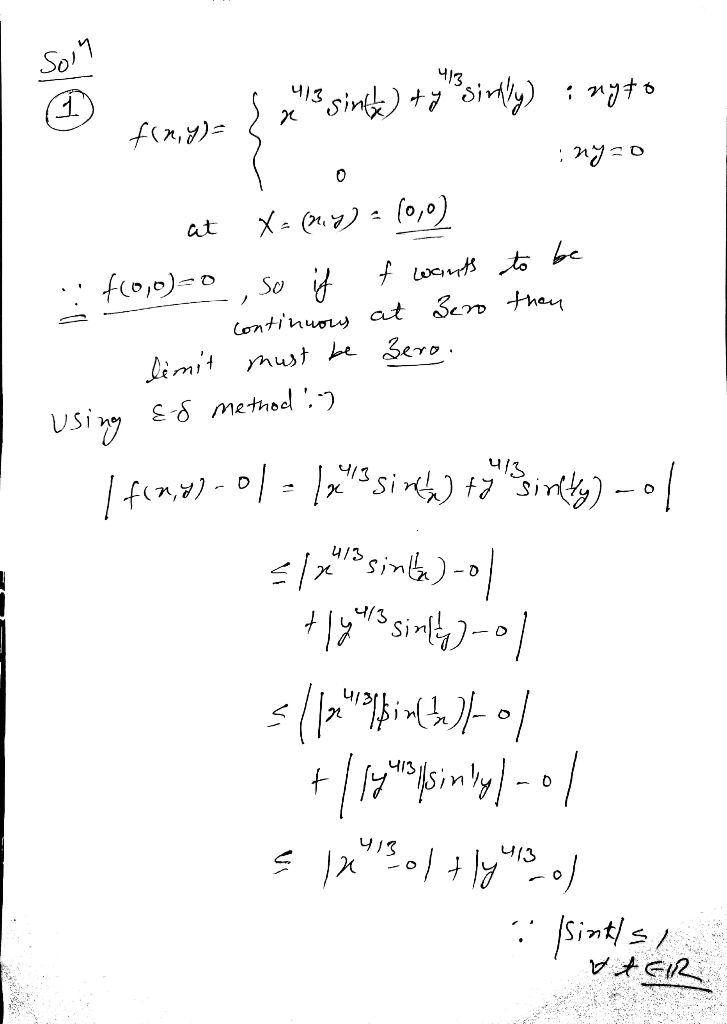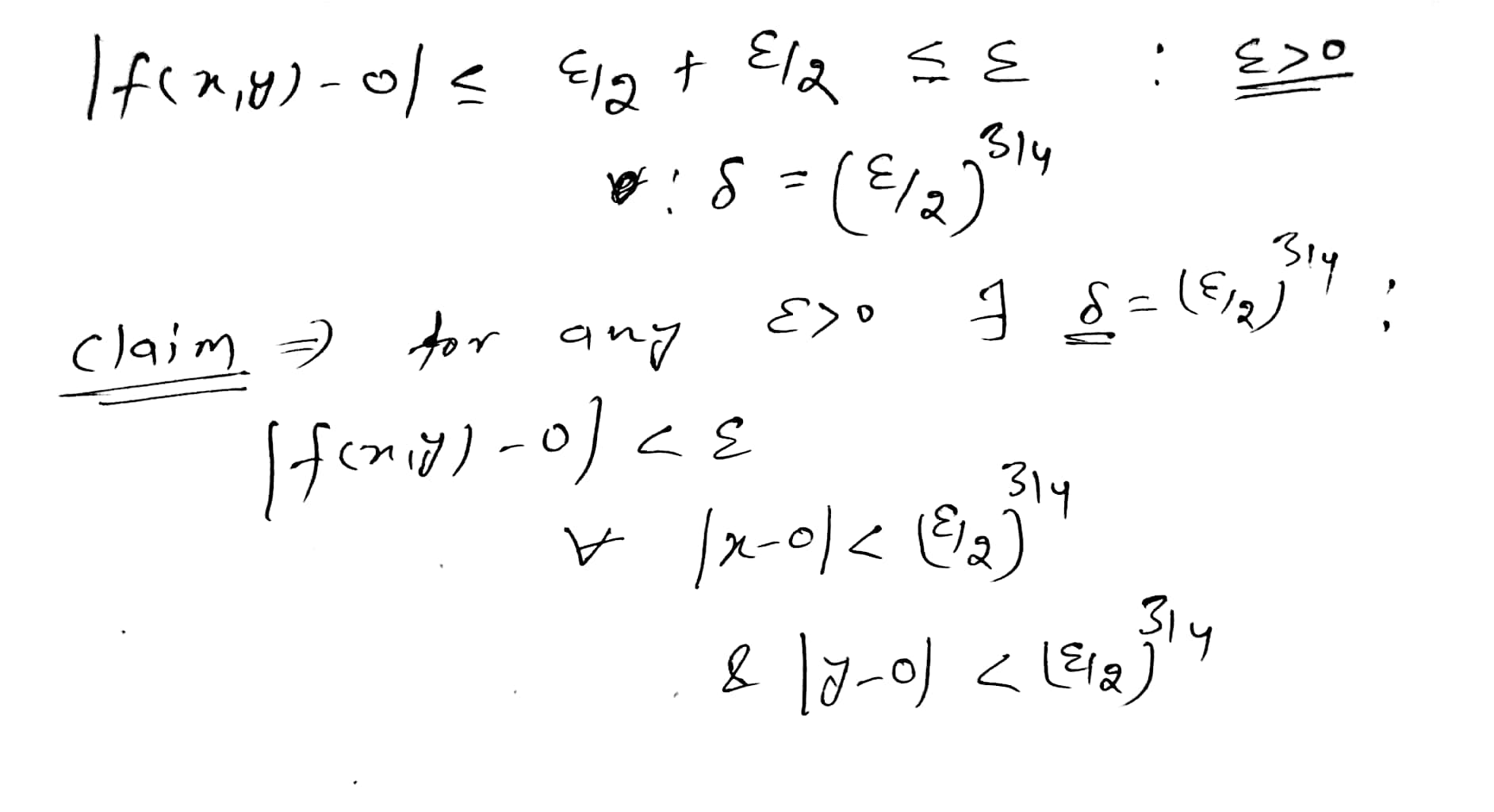#### Earn Coin

Coins can be redeemed for fabulous gifts.

Similar Homework Help Questions
• ### Exercice 2 (5pts) Let f given by f(x, y) Isinyif (x, y) (0,0) and f(0,0) 0...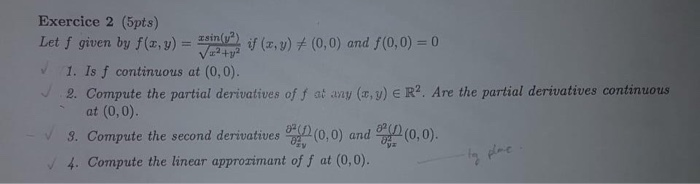Exercice 2 (5pts) Let f given by f(x, y) Isinyif (x, y) (0,0) and f(0,0) 0 1V224 1. Is f continuous at (0,0). 2. Compute the partial derivatives of f at any (x, y) E R2. Are the partial derivatives continuous (0,0). at (0,0) (0,0) and 3. Compute the second derivatives 4. Compute the linear approzimant of f at (0,0). Exercice 2 (5pts) Let f given by f(x, y) Isinyif (x, y) (0,0) and f(0,0) 0 1V224 1. Is f...

• ### Consider the function f: RR given by if (x, y)メ(0,0) íf (z, y) = (0,0). f(z,...Consider the function f: RR given by if (x, y)メ(0,0) íf (z, y) = (0,0). f(z, y)=(0+r R2 does the directional derivative Duf(0,0) exist? Evaluate the For which vectors 0メu directional derivative wherever it exists. 4 marks

• ### if (r.y) (0,0), 0,f (, y) (0, 0) 2. Consider f : IR2 -R defined by f(r,y)-+ (a) Show by explicit computation that the directional derivative exists at (x, y)- (0,0) for all oi rections u є R2 with 1...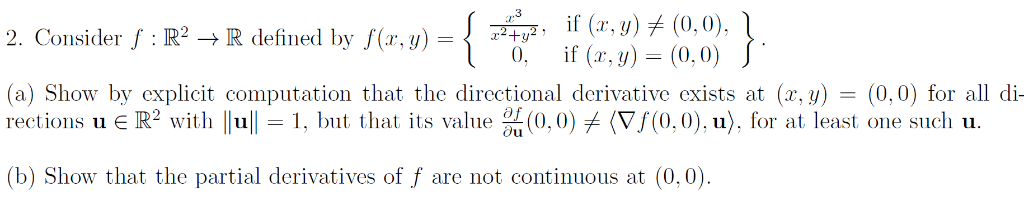if (r.y) (0,0), 0,f (, y) (0, 0) 2. Consider f : IR2 -R defined by f(r,y)-+ (a) Show by explicit computation that the directional derivative exists at (x, y)- (0,0) for all oi rections u є R2 with 1 11-1, but that its value %(0.0) (Vf(0,0).u), fr at least one sucli u. (b) Show that the partial derivatives of f are not continuous at (0,0) if (r.y) (0,0), 0,f (, y) (0, 0) 2. Consider f : IR2 -R...

• ### Anyone can solve these questions? (4) Let if (z, y)メ(0,0) if (x, y) (0,0) f(z, y)...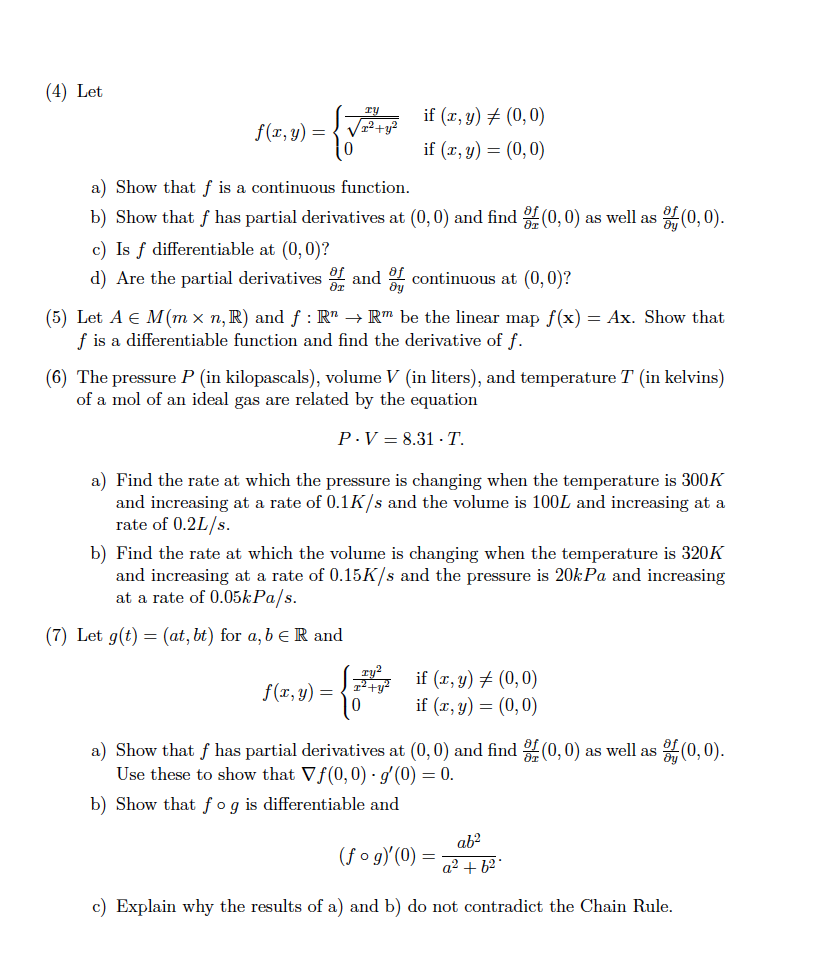Anyone can solve these questions? (4) Let if (z, y)メ(0,0) if (x, y) (0,0) f(z, y) / 0 a) Show that f is a continuous function b) Show that f has partial derivatives at (0,0) and find (0,0) as well as c) Is f differentiable at (0, 0)? d) Are the partial derivatives r tinuous at (0,0)? (0,0) (5) Let A E M(mx n,R) and f : RnRm be the linear map f(x)-Ax. Show that f is a differentiable function...

• ### = f ху Consider the function f(x,y) if x² + y² (x, y) + (0,0) =...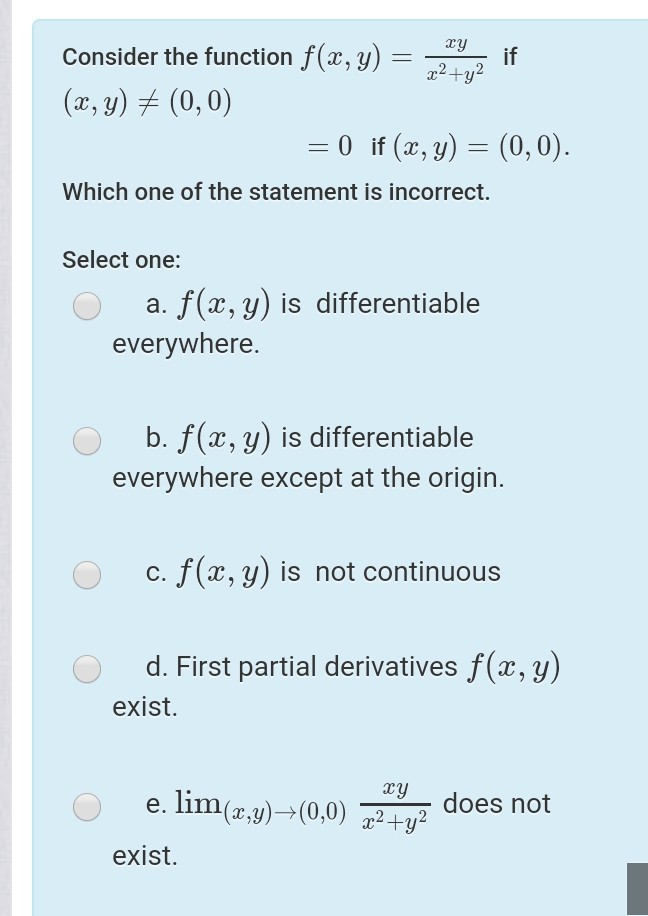= f ху Consider the function f(x,y) if x² + y² (x, y) + (0,0) = 0 if (x, y) = (0,0). Which one of the statement is incorrect. Select one: a. f(x,y) is differentiable everywhere. b. f(x,y) is differentiable everywhere except at the origin. c. f(x,y) is not continuous d. First partial derivatives f(x,y) exist. ху e. lim(x,y)—(0,0) x2 + y2 does not exist.

• ### 2. Consider the function f : R2 → R defined below. r3уг_ if (x,y) (0,0) f(x,y)...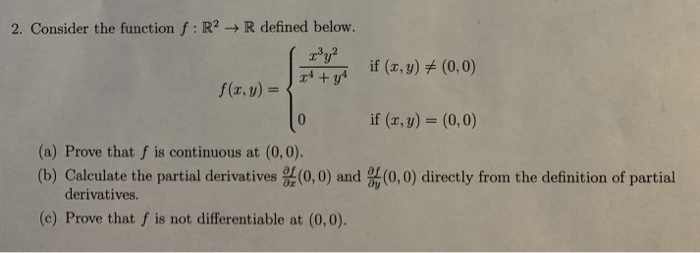2. Consider the function f : R2 → R defined below. r3уг_ if (x,y) (0,0) f(x,y) = if (x, y) (0, 0) (a) Prove that f is continuous at (0,0) (b) Calculate the partial derivatives (0,0) and (0,0) directly from the definition of partial derivatives. (c) Prove that f is not differentiable at (0,0).

• ### 3. Find lim f(,y) if it exists, and determine if f is continuous at (0,0. (x,y)--(0,0)...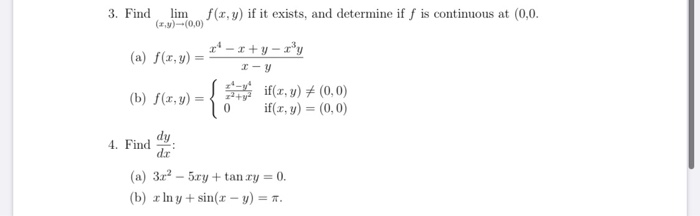3. Find lim f(,y) if it exists, and determine if f is continuous at (0,0. (x,y)--(0,0) (a) f(1,y) = (b) f(x,y) = { 0 1-y if(x, y) + (0,0) if(x,y) = (0,0) 4. Find y (a) 3.c- 5xy + tan xy = 0. (b) In y + sin(x - y) = 1.

• ### *Let f : R2 -R be given by z, y)(0,0 r, y)- 2y and f(0,0) =...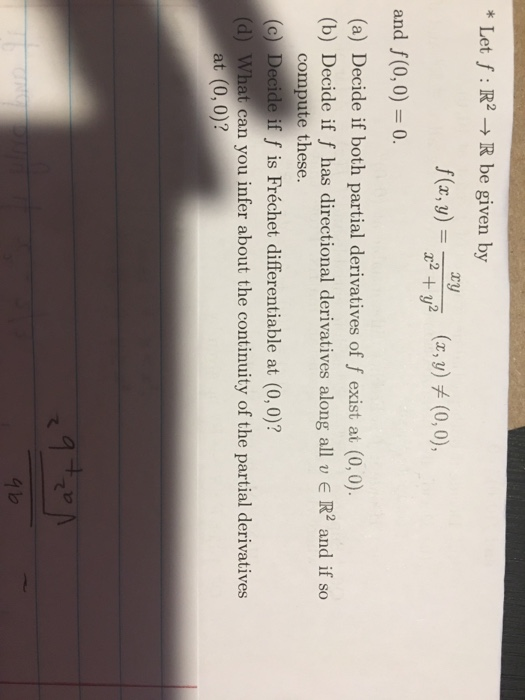*Let f : R2 -R be given by z, y)(0,0 r, y)- 2y and f(0,0) = 0. (a) Decide if both partial derivatives of f exist at (0, 0) (b) Decide if f has directional derivatives along all v R2 and if so compute these. (c) Decide if f is Fréchet differentiable at (0, 0)? (d) What can you infer about the continuity of the partial derivatives at (0, 0)? て

• ### Please answer fully and clearly. Thank you. )if (e-) (0.0)) if (z, y)#10, 0), 2. Consider f R2R defined by f(,) plHP, 0, if (x,y) (0,0)J (a) Show by explicit computation that the directional derivati...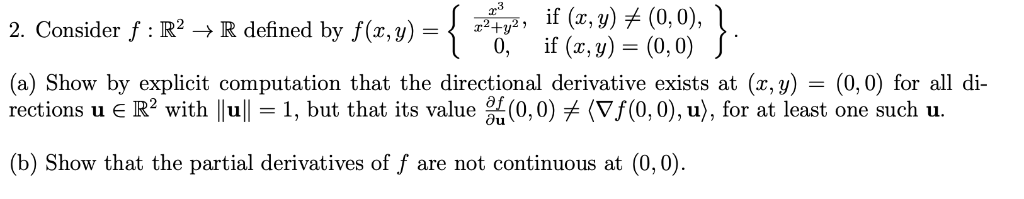Please answer fully and clearly. Thank you. )if (e-) (0.0)) if (z, y)#10, 0), 2. Consider f R2R defined by f(,) plHP, 0, if (x,y) (0,0)J (a) Show by explicit computation that the directional derivative exists at (x, y) - (0,0) for all di rections u R2 with lull-1, but that its value (0,0)メ(Vf(0,0), u), for at least one such u. b) Show that the partial derivatives of f are not continuous at (0,0) )if (e-) (0.0)) if (z, y)#10,...

• ### Let f(x,y) = (x" + 2?y?)!. compute all second-order partial derivatives of fat (0,0), if they...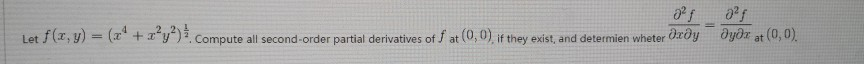Let f(x,y) = (x" + 2?y?)!. compute all second-order partial derivatives of fat (0,0), if they exist, and determien wheter dæðyəyər at (0,0).